# reappraised

The Reappraised package is a series of tools aiming to assess whether the distributions (and other aspects) of variables arising from randomisation in a group of randomised trials are consistent with distributions that would arise by chance. There are ten techniques described here.

# Contents

To start, the data set needs to be uploaded. The function load_clean() does this. It takes an excel spreadsheet or a data frame already in R and changes it into the format each function needs. It also checks for a few fundamental errors that would prevent the functions working and returns the data frame in a list. The description of what variables are needed and their format is in the help file for the function

eg Using the SI_pvals_cont data set:

 pval_cont_data <- load_clean(import= "no", file.cont = "SI_pvals_cont", pval_cont= "yes", format.cont = "wide")$pval_cont_data Or for an excel spreadsheet: pval_cont_data <- load_clean(import= "yes", pval_cont = "yes", dir = "path", file.name.cont = "filename", sheet.name.cont = "sheet name", range.name.cont = "range of cells", format.cont = "wide")$pval_cont_data

# get path for example files
path <- system.file("extdata", "reappraised_examples.xlsx",
package = "reappraised", mustWork = TRUE)
# delete file name from path
path <- sub("/[^/]+$", "", path) # load data pval_cont_data <- load_clean(import= "yes", pval_cont = "yes", dir = path, file.name.cont = "reappraised_examples.xlsx", sheet.name.cont = "SI_pvals_cont", range.name.cont = "A1:O51", format.cont = "wide")$pval_cont_data

One continuous and one categorical data set can be loaded in the same step but there are some limitations eg an excel spreadsheet and a R data frame can’t be loaded in the same step.

## Distribution of baseline p-values for continuous variables

P-values for the comparisons of baseline continuous variables will be uniformly distributed if the tests (t-test/anova etc) are done on raw data, but not if they are done on summary statistics (see Bolland et al, Baseline P value distributions in randomized trials were uniform for continuous but not categorical variables. J Clin Epidemiol 2019;112:67-76; and Bolland et al, Rounding, but not randomization method, non-normality, or correlation, affected baseline P-value distributions in randomized trials. J Clin Epidemiol 2019;110:50-62.)

To check the distribution, use the pval_cont_fn() to generate the distribution of p-values and the associated AUC of the ECDF.

First the distribution:

base_pval_cont <- pval_cont_fn(pval_cont_data, btsp= 500, verbose = FALSE)
base_pval_cont$all_results$pval_cont_calculated_pvalues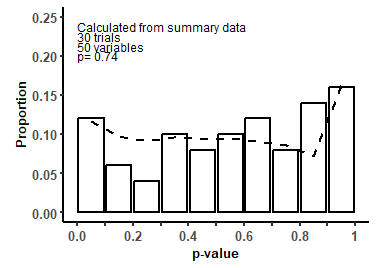Then the area under the curve ie the amount the distribution of values varies from the expected distribution:

base_pval_cont$all_results$pval_cont_auc_calculated_pvalues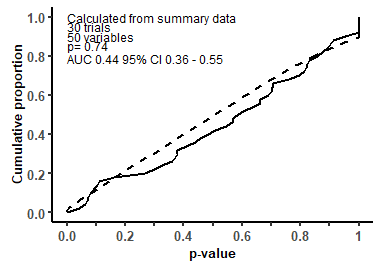For this example we can see that the distribution is consistent with the expected distribution, but 50 variables is a relatively small number and only a very large difference from the expected could be detected.

These p-values were calculated from the summary statistics so the expected distribution is not uniform, and the expected ECDF is not a straight line. If you use reported p-values (presumably calculated from the raw data), then the expected distribution is uniform and the expected distribution in the ECDF a straight line.

base_pval_cont$all_results$pval_cont_reported_pvalues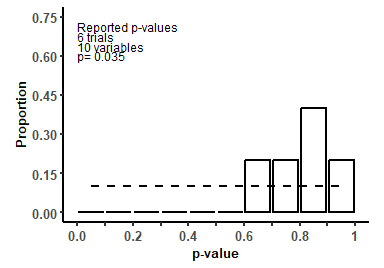base_pval_cont$all_results$pval_cont_auc_reported_pvalues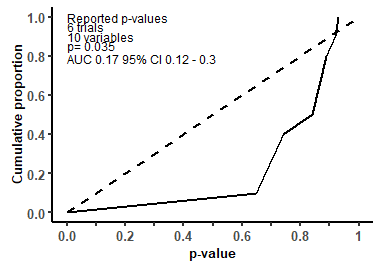In this case, the distribution is not consistent with expected, and the AUC differs markedly from the expected value of 0.5. However: there are not many reported p-values in this example and so no reliable conclusions can be drawn.

## Matches of baseline summary statistics

Matching baseline summary statistics (means and SDs) in randomised trials occur but are uncommon. In two-arm trials, the situation where the mean for a variable is identical in both groups AND the SD for that variable is also identical in both groups is very uncommon. Variables with fewer significant figures and variables with small SDs are likely to have higher matching means or matching SDs or both a matching mean and a matching SD. (See Bolland et al. Identical summary statistics were uncommon in randomized trials and cohort studies. J Clin Epidemiol 2021;136:180-188.) Reference data from a large database of trials collected by John Carlisle are described in the article.

To check the frequency of matching summary statistics, use the match_fn() to generate a summary table with a comparison to the reference data:

match_data <- load_clean(import= "no", file.cont = "SI_pvals_cont", match= "yes", format.cont = "wide", verbose = FALSE)$match_data match_fn(match_data, verbose= FALSE)$match_ft_all

Variable

All variables

1 Sig. dig.

2 Sig. dig.

3 Sig. dig.

4 Sig. dig.

5 Sig. dig.

27 trials

Number of variables, n (%)

Mean

46

1 (2.2)

15 (32.6)

25 (54.3)

5 (10.9)

SD

46

5 (10.9)

32 (69.6)

9 (19.6)

0 (0.0)

Proportion of matching summary statistics in both treatment groups (%)

Means match

10.9

0.0

20.0

8.0

0.0

SDs match

10.9

20.0

12.5

0.0

Both Mean and SD matches

2.2

0.0

3.2

0.0

Reference data

3585 trials

Number of variables, n (%)

Mean

21948

607 (2.8)

8149 (37.1)

10978 (50.0)

2082 (9.5)

132 (0.6)

SD

21145

4320 (20.4)

10682 (50.5)

5618 (26.6)

552 (2.6)

36 (0.2)

Proportion of matching summary statistics in both treatment groups (%)

Means match

13.4

66.4

20.6

7.5

1.6

0.8

SDs match

14.8

40.5

11.6

2.7

0.4

0.0

Both Mean and SD matches

5.1

16.9

2.7

0.2

0.0

0.0

The table shows that the number of matches is similar to the reference data set but conclusions are limited by the relatively small number of variables.

## Matches of summary statistics in different cohorts

Similar to matching summary statistics within a trial, it is uncommon for identical summary statistics to be reported in different trials from the same population group. For example, if two separate trials are recruited in patients with osteoporosis, it would be unusual for their age to be identical. (See Bolland et al. Identical summary statistics were uncommon in randomized trials and cohort studies. J Clin Epidemiol 2021;136:180-188.)

To check the frequency of matching summary statistics across different cohorts, use the cohort_fn() to generate a summary table…

cohort_data <- load_clean(import= "no", file.cont = "SI_cohort", cohort= "yes", format.cont = "long", verbose= FALSE)$cohort_data cohort <- cohort_fn(cohort_data, seed = 10, sims = 100, verbose= FALSE) cohort$cohort_ft

Mean

SD

Both Mean and SD

Variable

Mean
(N)a

Mean Match
N (%)a

Largest Number Mean Matches
N (%)a

P Mean Matchc

SD
(N)a

SD Match
N (%)a

Largest Number SD Matches
N (%)a

P SD Matchc

Mean (SD)
Mode or Averageb

Mean and SD Match
N (%)b

Largest Number Mean and SD Matches
N (%)a

P Mean and SD Matchc

Age

10

0 (0)

0 (0)

0.1

9

0 (0)

0 (0)

0.12

73.2 (4.1)

0 (0)

0 (0)

0.89

BMI

10

0 (0)

0 (0)

0.01

10

3 (30)

3 (30)

0.01

22.3 (2)

0 (0)

0 (0)

0.24

ICTP

10

3 (30)

3 (30)

0.17

9

4 (44)

4 (44)

0.08

7.1 (1.1)

3 (30)

3 (30)

0.19

Osteocalcin/Bone Gla (BGA)

10

2 (20)

2 (20)

0.42

9

4 (44)

4 (44)

0.44

7 (4.5)

2 (20)

2 (20)

0.12

BMD

10

2 (20)

2 (20)

0.68

9

2 (22)

2 (22)

0.44

2.55 (0.36)

2 (20)

2 (20)

0.07

iCa (mmol/L)

10

8 (80)

4 (40)

0.54

10

6 (60)

2 (20)

0.19

1.239 (0.047)

0 (0)

0 (0)

0.02

Dietary vitamin D

10

6 (60)

2 (20)

0.54

10

6 (60)

2 (20)

0.35

124 (25)

6 (60)

2 (20)

0.01

PTH

10

4 (40)

2 (20)

0.52

8

4 (50)

2 (25)

0.17

33.12 (8.9)

4 (40)

2 (20)

0.01

1,25(OH)2Db

10

6 (60)

4 (40)

0.04

10

5 (50)

3 (30)

0.13

49.6 (9.2)

3 (30)

3 (30)

0.01

25(OH)Da

10

4 (40)

2 (20)

0.42

10

4 (40)

2 (20)

0.66

25 (5.8)

4 (40)

2 (20)

0.01

aThe columns represent the number of reported means and SD; the number of means, SD, and both mean and SD that match amongst the different cohorts; and the largest number of means, SD, or both matching mean and SD in a single cohort that had a matching value in a different cohort

bwhere there was no matching mean (SD), the average mean and SD in the cohorts weighted by participant are reported, otherwise the most common mean (SD), ie the mode, is reported together with the number of occurrences of the mode

cP refers to the probability of the reported number of matching means, matching SDs or both matching mean and matching SD for each variable in 100 simulations

In this example, 1,25OHD (3), 25OHD (4), Dietary vitamin D (6), PTH (4) all had a higher number of matches for the combination of mean/SD in the 10 cohorts than expected (and iCa had a lower number than expected)

… Or use cohort_fn() to generate a graph of the observed to expected numbers of matches per cohort.

cohort$cohort_all_graphs$all_graph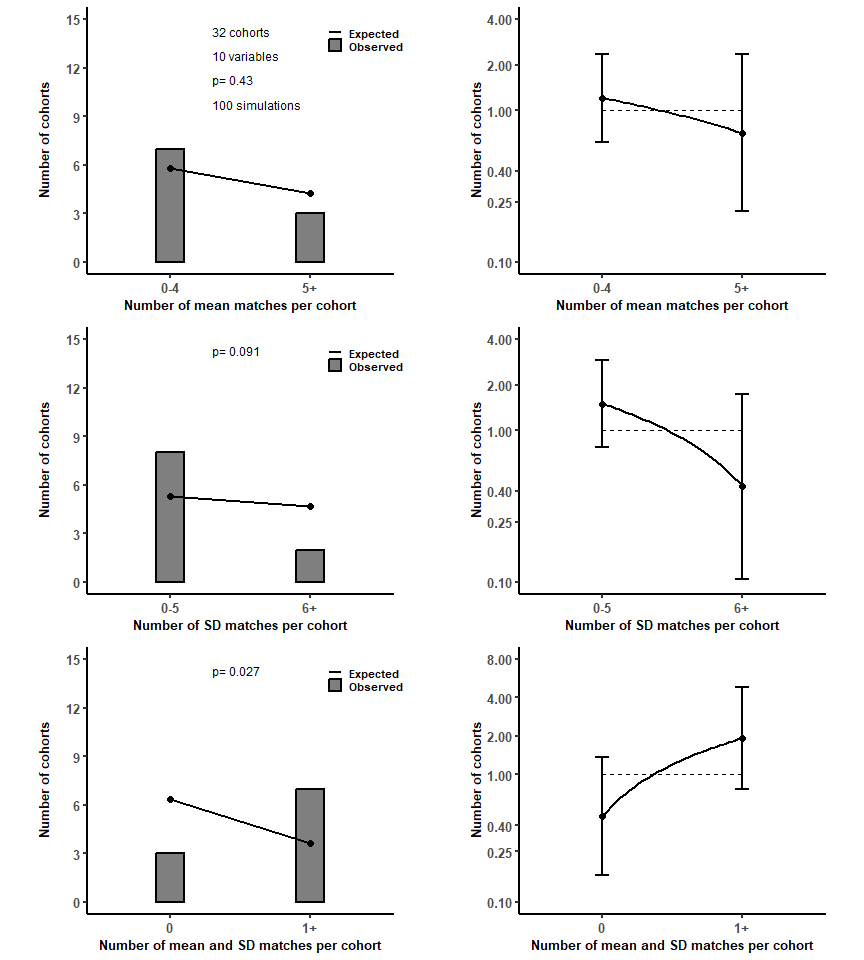In this example, there are 3 cohorts with 0 matching mean/SD combination compared with the expected value of 6.2; and 7 cohorts with 1 or more matching mean/SD combinations compared with the expected value of 3.8. Although there are only 10 cohorts, this is still an improbable result.

## Comparing means between groups

To compare the distribution of p-values for differences in baseline means calculated using Carlisle’s montecarlo anova method, use anova_fn(). (Method is from Carlisle JB, Loadsman JA. Evidence for non-random sampling in randomised, controlled trials by Yuhji Saitoh. Anaesthesia. 2017;72:17-27. R code is in appendix to paper.)


anova_data <- load_clean(import= "no", file.cont = "SI_pvals_cont",anova= "yes", format.cont = "wide")$anova_data anova_fn(anova_data, seed = 10, sims = 100, btsp = 100, verbose = FALSE)$anova_ecdf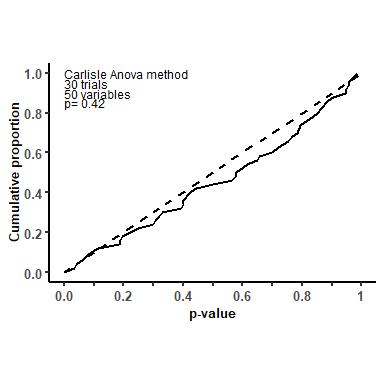In this case, the distribution of p-values are consistent with the expected distribution.

There are two methods. method = 'orig' adapted from the code published by Carlisle, which uses a row-wise approach and several loops. For Carlisle’s code, data in the long form was required, and the code seemed to go slowly. So I developed method = 'alt' which does all the simulations in one step and uses a lot more memory but is much faster than the original code. However, when the row-wise approach is used on data in wide format, the speed benefit from the alternate method is marginal. For large simulations, it is worth experimenting to see how much memory is required and what speed trade off there is.

## Distribution of a single categorical variable

The expected distribution of baseline categorical variables (or outcome variables unrelated to treatment allocation) is the binomial distribution. Therefore the observed distribution of variables with 0, 1, 2, 3 … participants in each treatment group, and the differences between the randomised groups in two-arm studies, can be compared with the expected distributions. (Bolland et al Participant withdrawals were unusually distributed in randomized trials with integrity concerns: a statistical investigation. J Clin Epidemiol 2021;131:22-29.)

To compare the observed and expected distributions, use the cat_fn() to generate a graph of observed to expected frequencies

cat_data <- load_clean(import= "no", file.cat = "SI_cat", cat= "yes", format.cat = "wide", cat.names = c("n", "w"))$cat_data cat_fn(cat_data, x_title="withdrawals", prefix="w", del.disparate="yes", verbose = FALSE)$cat_graph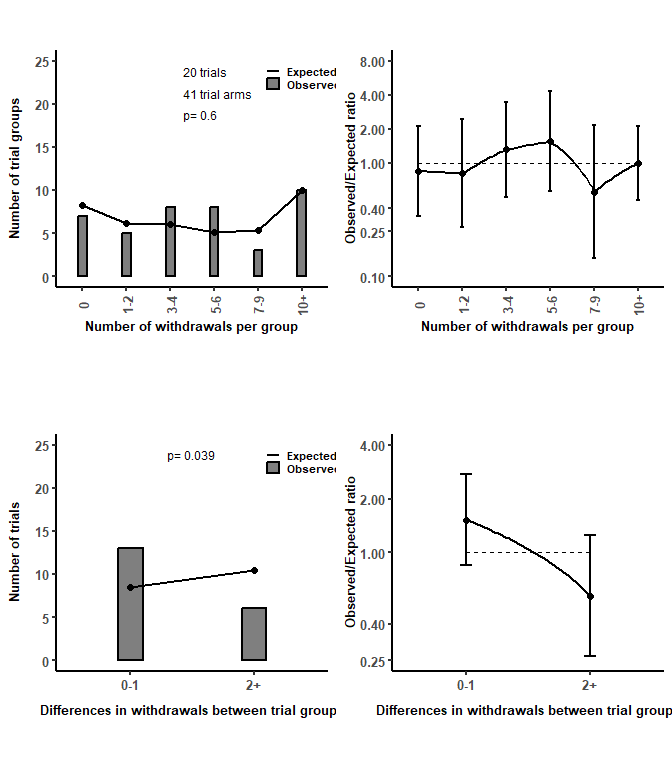The plots show more differences in withdrawals of 0 or 1 between the trials groups than expected and fewer differences in withdrawals of 2 or more than expected.

## Distributions of numbers of participants in trial groups

The same principle applies for the number of participants in trials as for a single categorical variable. If simple randomisation has occurred, the expected distribution of the number of participants, and the differences between groups in two arm trials, is the binomial distribution. An example is in Bolland et al. Systematic review and statistical analysis of the integrity of 33 randomized controlled trials. Neurology 2016;87:2391-2402.

To compare the observed and expected distributions of trial participants in trials using simple randomisation, use the sr_fn() which generates a graph of observed to expected frequencies

sr_data <- load_clean(import= "no", file.cat = "SI_cat", sr= "yes", format.cat = "wide")$sr_data sr_fn(sr_data, verbose = FALSE)$sr_graph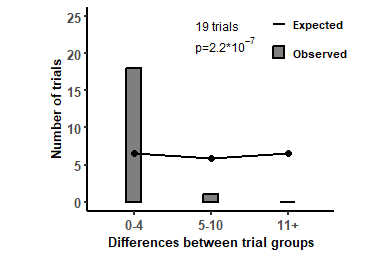The figure shows too many trials in which the participant numbers were similar (difference 0-4) in each group if simple randomisation occurred.

If a trial uses block randomisation, and the final block size is known, then the number of participants in the final block can be determined. For pragmatic reasons, you’d expect the final block to be filled (since the pre-planned number of participants are recruited). But if the final block number can be determined, the distribution between groups for the final block numbers can be determined. However, it seems unlikely that many studies would provide sufficient data to do this.

If data are available, use the the block randomisation options in sr_fn()

#create data set
block = data.frame(study = c(1,2,3,4,5,6,7,8,9,10), #study numbers
fb_sz= c(2,4,6,8,10,12,8,8,6,14), #final block size
n_fb = c(1,1,4,5,7,8,4,6,2,10), #number in final block
df=c(1,1,0,1,3,4,2,2,0,0)) #difference between groups

cat_all_fn (cat_all_data, binom = "yes", two_levels = "y", del.disparate = "y", excl.level = "yes", seed = 10, verbose = FALSE)$cat_all_graph_pc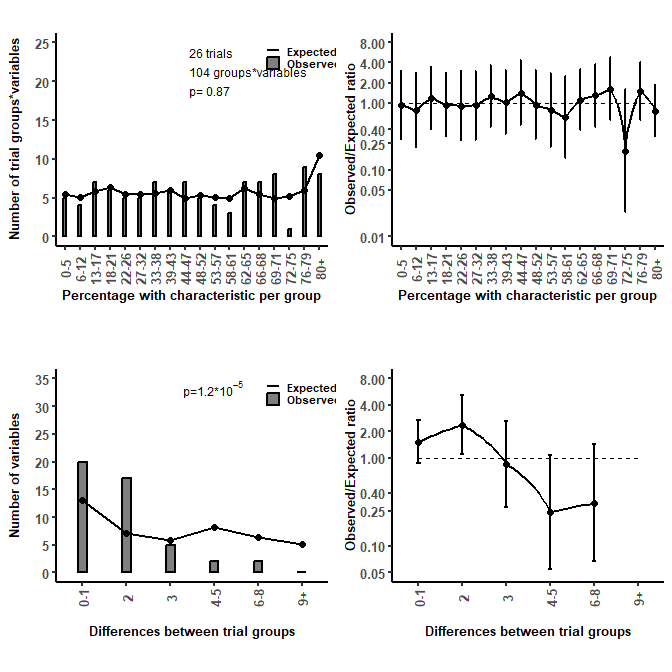In this example, there are more variables with a difference of 0-1, or 2 between the groups, and fewer variables with a difference of >4 compared to the expected distribution. If variables have more than 2 levels (eg brown eyes, blue eyes, green eyes), using two_levels = "y" recodes the data into the two largest groups. Since for each variable with k levels, there are only k-1 independent levels (the final level can be calculated from the total - sum of other levels), double counting can occur. excl.level = "yes" excludes a randomly selected level from the analysis. ## Comparing reported and calculated p-values for categorical variables As a p-value for the comparison of a categorical variable between groups (eg using Chi-square or Fisher’s exact test) can be calculated from the summary statistics, reported p-values can be compared with independently calculated values. (See Bolland et al. Distributions of baseline categorical variables were different from the expected distributions in randomized trials with integrity concerns. J Clin Epidemiol. 2023;154:117-124) To compare reported and calculated p-values, use the cat_all_fn() to produce a table of the comparison. cat_all_fn (cat_all_data, comp.pvals = "yes", verbose= FALSE)$cat_all_diff_calc_rep_ft

Test

Baseline p-value

Difference between
reported and
calculated p-values

n (%)

Chisq

Fisher

0.5 - 0.6

0.6 - 0.7

0.7 - 0.8

0.8 - 0.9

0.9 - 1

0

17 (68)

16

1

1

5

5

5

1

0 - 0.1

6 (24)

6

1

1

3

1

0.1 - 0.2

1 (4)

1

1

0.2 - 0.3

1 (4)

1

1

0.3 - 0.4

0.4 - 0.5

0.5 - 0.6

0.6 - 0.7

0.7 - 0.8

0.8 - 0.9

0.9 - 1

In this example (from a real data set of trials), all the calculated p-values were >0.5 and 2 differed by >0.1 from the reported value.

## Distributions of p-values for categorical variables

Although the distributions of p-values from the comparison between groups for categorical variables are not uniform, they can be empirically calculated. (Bolland et al. Baseline P value distributions in randomized trials were uniform for continuous but not categorical variables. J Clin Epidemiol 2019;112:67-76.)

To compare the observed and expected distribution, use the pval_cat_fn() to generate the distribution of p-values and the associated AUC of the ECDF. See pval_cont_fn() for more details.


pval_cat_data <- load_clean(import= "no", file.cat = "SI_cat_all", pval_cat= "yes", format.cont = "wide", verbose = FALSE)$pval_cat_data pval_cat_fn(pval_cat_data, sims = 10, seed=10, btsp = 100, verbose = FALSE)$pval_cat_calculated_pvalues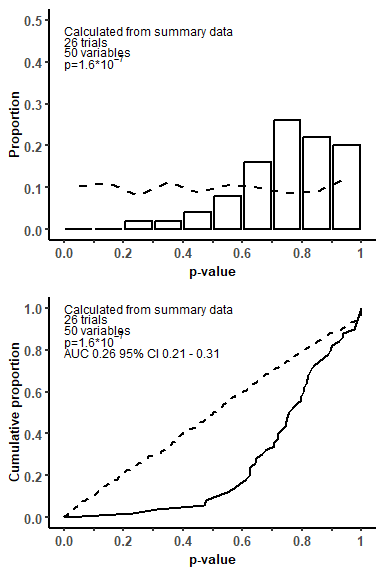In this example, the observed distribution differs markedly from the expected distribution.

The help file gives some details of the methods. You can choose to calculate p-values with Chi-square or Fisher’s exact test (stat = 'chisq' or stat = 'fisher'), or override the tests given in the data frame stat.overide = yes. method describes the analysis: method = 'sm' tests on summary data; method = 'ind' tests done on individual data; and method = 'mix' uses individual data for Fisher’s exact and summary data for Chi-square. If there are a large number of simulations, it might be worth comparing the duration of the various methods with your data frame and a small number of simulations before running the large simulation.

## Distribution of final digits

This is an approach in development and requires further use and validation. The frequency of final digits of the summary statistics for a group of variables is plotted.


generic_data <- load_clean(import= "No", file.cont = "SI_pvals_cont", generic= "yes", gen.vars.del = c("p"), format.cont = "wide")$generic_data final_digit_fn(generic_data, vars = c("m","s"), dec.pl = "n", verbose = FALSE)$digit_graph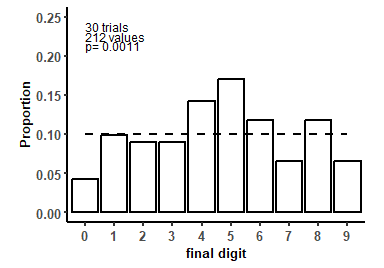This example shows that there are more summary statistics ending in 4,5 and fewer ending in 9,0. One difficulty is that R and excel drop trailing 0s from numbers so to record a value of 1.0 it needs to be stored as character/text. But in almost all situations, numbers are not recorded or saved as text. To get round this, the number of decimal places for a variable is assumed to be constant across treatment groups and the maximum number of decimal places used.

As many variables will be rounded, it is difficult to know what the expected distribution of final digits should be. In Carlisle’s dataset of >5000 trials and 140000 variables, the distribution was approximately uniform except there were fewer 0’s.

## Final comment

If you use the package or any of the functions, let me know. I’d be interested to hear about the results. Plus if there are errors anywhere, or things I can do to improve the package, or other functions to add in, please do let me know. I’m largely self-taught in R, and I’m sure the code and package can be improved.